# 【画学numpy】2.numpy数组创建

⭐️github：drawing_and_studying_numpy包含了可以运行的notebook文件以及本文绘制的所有插图。

🌈画学numpy系列文章目录：

# numpy数组创建

• 等间隔数组：数组两两之间间隔相同，类似等差数列。
• 全等数组：数组里所有元素都相同。
• 随机数组：按要求随机生成数组，做模拟的时候很有用。
[外链图片转存失败,源站可能有防盗链机制,建议将图片保存下来直接上传(img-uz8DiaYd-1647971377746)(./images/2.numpy数组创建_思维导图1.png)]
``````import numpy as np
print(np.__version__)
``````
``````1.22.3
``````

## 等间隔数组

• `np.arange()`: 按照给定的 步长根据起点和终点生成一维数组
• `np.linspace()`: 按照给定的 数组长度，均匀划分起点到终点的距离生成一维数组

### np.arange()

📖官方文档

``````numpy.arange(start, end, step, ...)
``````

`np.arange()`函数和python自带的 `range()`函数十分类似。

• `start`: 起点，默认为0
• `end`: 终点
• `step`: 步长，默认为1``````a = np.arange(4)
print(a)
``````
``````[0 1 2 3]
````````````np.arange(2, 6)
``````
``````array([2, 3, 4, 5])
````````````np.arange(2, 9, 2)
``````
``````array([2, 4, 6, 8])
``````

`range`的一大区别是 `np.arange`的步长可以是小数``````np.arange(1, 1.4, 0.1)
``````
``````array([1. , 1.1, 1.2, 1.3])
``````

### np.linspace()

📖官方文档

``````numpy.linspace(start, end, num=50, ...)
``````
• `start`: 起点
• `end`: 终点
• `num`: 一维数组长度

`num=5`时，生成的数组长度为5，生成的数组包含了起点1和终点4，且前后两个元素的间隔相等``````np.linspace(1, 4, num=5)
``````
``````array([1.  , 1.75, 2.5 , 3.25, 4.  ])
``````

`num=4```````np.linspace(1, 4, num=4)
``````
``````array([1., 2., 3., 4.])
``````

`num=3```````np.linspace(1, 4, num=3)
``````
``````array([1. , 2.5, 4. ])
``````

`num=2`时，生成的数组恰好是由起点和终点组成的``````np.linspace(1, 4, num=2)
``````
``````array([1., 4.])
``````

`num=1`时，生成了一个只有起点的数组``````np.linspace(1, 4, num=1)
``````
``````array([1.])
``````

## 全等数组

• `np.zeros()`: 生成一个全为0的一维或多维数组。
• `np.ones()`: 生成一个全为1的一维或多维数组。
• `np.full()`: 生成一个元素全部相等的一维或多维数组。

### np.zeros()

📖官方文档

``````numpy.zeros(shape, ...)
``````

``````np.zeros(shape=4)
``````
``````array([0., 0., 0., 0.])
``````

``````np.zeros(shape=(3, 4))
``````
``````array([[0., 0., 0., 0.],
[0., 0., 0., 0.],
[0., 0., 0., 0.]])
``````### np.ones()

📖官方文档

``````numpy.ones(shape, ...)
``````

``````np.ones(shape=4)
``````
``````array([1., 1., 1., 1.])
``````

``````np.ones(shape=(3, 4))
``````
``````array([[1., 1., 1., 1.],
[1., 1., 1., 1.],
[1., 1., 1., 1.]])
``````### np.full()

📖官方文档

``````numpy.full(shape, fill_value, ...)
``````

• shape: 数组的形状
• fill_value: 数组的值
生成一个全为2.4的一维数组
``````np.full(shape=4, fill_value=2.4)
``````
``````array([2.4, 2.4, 2.4, 2.4])
``````

``````np.full(shape=(3, 4), fill_value='a')
``````
``````array([['a', 'a', 'a', 'a'],
['a', 'a', 'a', 'a'],
['a', 'a', 'a', 'a']], dtype='<u1') < code></u1')>
``````## 随机数组

• `np.random.random()`: 生成一个 0到1之间均匀分布的随机数组
• `np.random.uniform()`: 生成一个 指定区间均匀分布的随机数组
• `np.random.randn()`: 生成一个 标准正态分布的的随机数组
• `np.random.normal()`: 生成一个 指定正态分布的的随机数组
• `np.random.randint()`: 生成一个指定区间范围的随机整数数组

### np.random.random()

📖官方文档

``````numpy.random.random(size)
``````

• size: 数组的形状

``````np.random.random(4)
``````
``````array([0.9155639 , 0.0879231 , 0.50418975, 0.79625964])
``````

``````np.random.random((3, 4))
``````
``````array([[0.29551936, 0.42407484, 0.65833207, 0.54675449],
[0.64280259, 0.42581316, 0.12087349, 0.72347867],
[0.36625864, 0.71585893, 0.24240265, 0.90152112]])
``````

### np.random.uniform()

📖官方文档

``````numpy.random.uniform(low=0.0, high=1.0, size=None)
``````

• `low`: 数组的最小值
• `high`: 数组的最大值
• `size`: 数组的形状

``````np.random.uniform(low=2, high=5, size=4)
``````
``````array([4.46704977, 3.28453679, 3.8914482 , 4.23255661])
``````

``````np.random.uniform(-3, 3, (3, 4))
``````
``````array([[ 0.53919671,  2.70351516,  1.91513643, -0.0198369 ],
[ 2.43241932, -2.99126798, -2.1072604 , -1.95992406],
[ 2.23506767, -2.32354201,  1.18681695, -0.09019135]])
``````

### np.random.randn()

📖官方文档

``````numpy.random.randn(d0, d1, d2, ...)
``````

• `d0, d1, ...`: 数组的形状
生成一个1维标准正态分布随机数组
``````np.random.randn(4)
``````
``````array([-0.97841294,  0.08917692,  0.05429765,  0.38660857])
``````

``````np.random.randn(3, 4)
``````
``````array([[ 1.64886552,  0.36417621,  0.77745513, -1.14481688],
[-1.0317714 , -0.13213342, -0.38355106, -1.09655604],
[ 1.5982574 ,  0.10802592, -1.94052385,  1.07061462]])
``````

### np.random.normal()

📖官方文档

``````numpy.random.normal(loc=0.0, scale=1.0, size=None)
``````

• `loc`: 数组的均值
• `scale`: 数组的标准差
• `size`: 数组的形状
生成一个均值为2，标准差为10的一维正态分布随机数组
``````np.random.normal(loc=2, scale=10, size=4)
``````
``````array([ 11.44176805, -18.58578423,  -0.1879512 ,  10.43410251])
``````

``````np.random.normal(-5, 5, size=(3, 4))
``````
``````array([[  0.78720712,  -1.01289235,  -2.97885233,   0.42613163],
[ -6.00490185,  -0.71370871,   0.1223361 ,  -9.68017774],
[ -3.09613496, -10.65665833,  -3.89814507,  -0.69998495]])
``````

### np.random.randint()

📖官方文档

``````numpy.random.randint(low, high=None, size=None, ...)
``````

• `low`: 最小值
• `high`: 最大值，如果 `high=None`，那默认生成的范围是 `[0, low)`。否则生成的范围是 `[low, high)`
• `size`: 数组的形状
生成一个0到5(不包含5)之间的随机一维整数数组
``````
np.random.randint(5, size=8)
``````
``````array([0, 1, 3, 4, 3, 0, 2, 2])
``````

``````np.random.randint(1, 8, (3, 4))
``````
``````array([[2, 6, 7, 4],
[3, 2, 5, 2],
[3, 4, 1, 4]])
``````

## 小结Original: https://blog.csdn.net/Light2077/article/details/123676032
Author: Light2077
Title: 【画学numpy】2.numpy数组创建

# 1、数据来源——Wind金融终端

(需要数据进行操作的同学可以在我的资源上下载，由于Wind资讯金融终端不是免费的，而且作为喜欢白嫖的我们，有什么办法得到免费的数据呢，下次找到了一定分享给大家zll[doge]）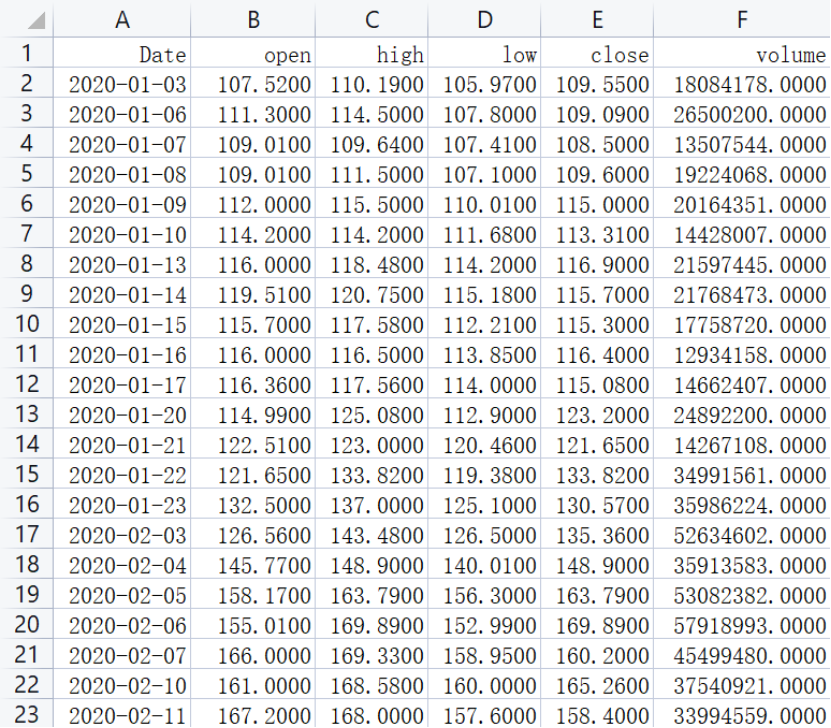# ; 2、数据读取及其基本描述

``````import pandas as pd
import matplotlib.pyplot as plt
``````

``````df = pd.DataFrame(data)
``````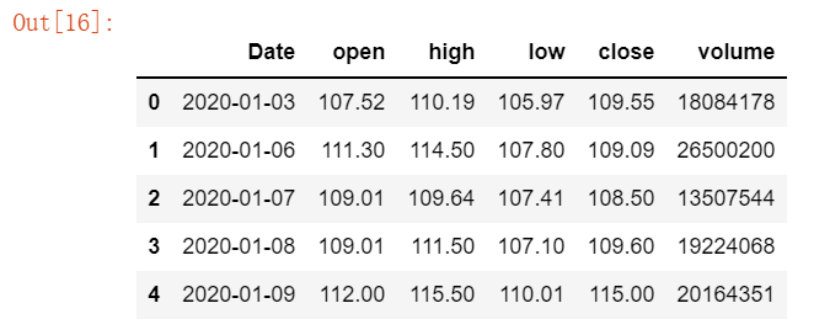``````df.describe()
``````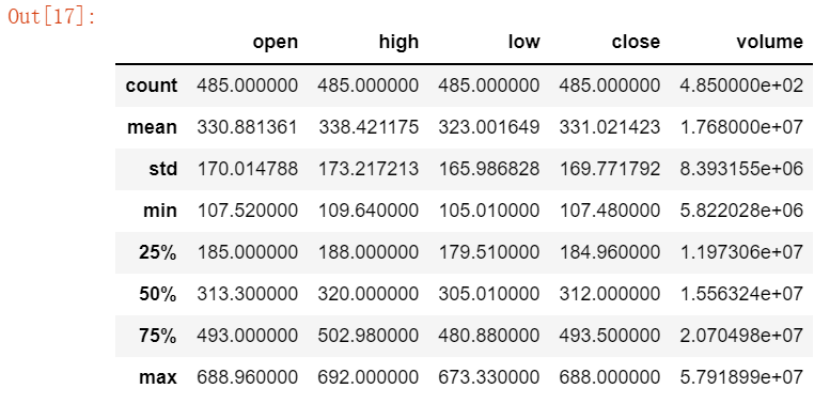# 3、收盘价与成交量关系图

``````
df['close'].plot()
``````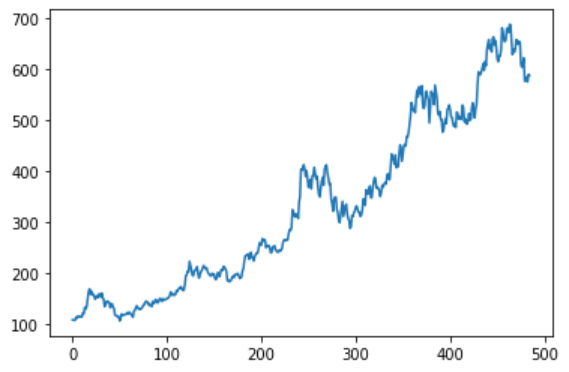``````
df[['close','volume']].plot(subplots=True)
``````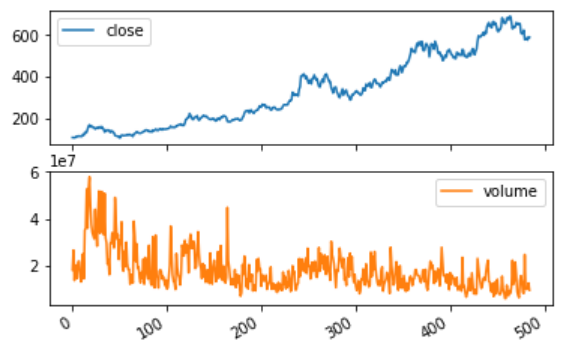``````list_date = list(df['Date'])
df.index = list_date

price = df['close']
volumes = df['volume']
top = plt.subplot2grid((4,4), (0,0), rowspan=3, colspan=4)
top.plot(price.index, price, label='close')
plt.title('300750.SZ Close Price from 2020-2021')
plt.legend(loc=2)

bottom = plt.subplot2grid((4,4), (3,0), rowspan=1, colspan=4)
bottom.bar(volumes.index, volumes)

plt.gcf().set_size_inches(12,8)
``````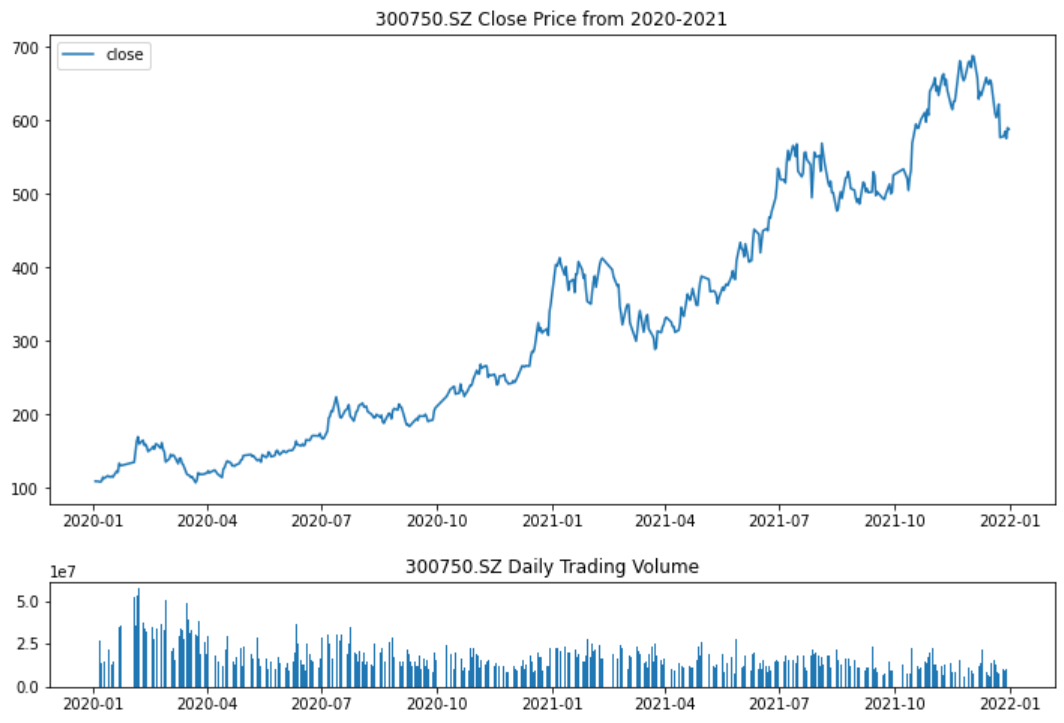# 4、收益率曲线

（>......

Original: https://blog.csdn.net/weixin_47974364/article/details/123408886
Author: 貮叁
Title: python金融数据分析及可视化

## 机器学习：SOM聚类的实现

SOM SOM算法是一种无监督学习的神经网络算法。由输入层和竞争层（输出层）组成。SOM是一种聚类方法。 ; 算法步骤 初始化竞争层；竞争层一行代表一个坐标点（x，y）。 根据竞争层的尺寸初始化权重矩...

## 浅谈点云与三维重建

《浅谈点云与三维重建》 【本期导读】 三维重建技术在各领域已经展现出了不可替代性，而点云作为三维重建的重要工具，却常常被忽略。本文将从概念定义、数据来源、类别划分、应用场景等方面来介绍点云并剖析点云是...